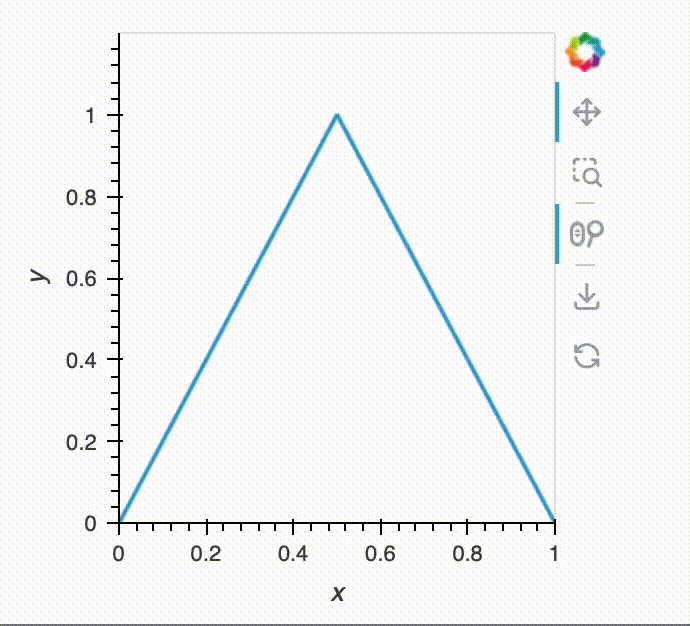# Auto-ranging y-axis on a DynamicMap with RangeX

I understood that I can use `framewise=True` on a `DynamicMap` to auto-range axes. And, for a simple case (see code below), this works.

However, the this does not seem to work for the case of `RangeX` stream. How can I autoscale y-axis in this case?

I am on holoviews 1.16.2.``````import holoviews as hv
hv.extension("bokeh")
import panel as pn

def simple_function(x_range):
if isinstance(x_range, tuple):
# for RangeX
x0,x1 = x_range
else:
# for the slider
x0 = x_range
x1 = 1-x0
dx = x1-x0
x = [x0,(x0+x1)/2,x1]
y = [0,1/dx,0]
c = hv.Curve((x,y))
c.opts(ylim=(0, 1.2/dx))
return c

# simple case with a slider
slider = pn.widgets.FloatSlider(value=0,start=0,end=0.5,step=0.01)
dm = hv.DynamicMap(pn.bind(simple_function, slider))
dm.opts(framewise=True)
pn.Column(slider, dm)
# works

# with RangeX
dm = hv.DynamicMap(
simple_function,
streams=[hv.streams.RangeX(x_range=(0,1))]
)
dm.opts(framewise=True) # does not solve
dm
``````